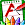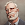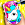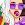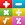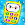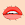# Math Games, Learn Add, Subtract, Multiply & Divide

by GunjanApps Studios
50,000,000+ installs

Android application Math Games, Learn Add, Subtract, Multiply & Divide developed by is listed under category Game Educational. According to Google Play Math Games, Learn Add, Subtract, Multiply & Divide achieved more than 50,000,000 installs. Math Games, Learn Add, Subtract, Multiply & Divide currently has 258,362 ratings with average rating value of 4.51. The current percentage of ratings achieved in last 30 days is 1.49%, percentage of ratings achieved in last 60 days is 3.27%. Math Games, Learn Add, Subtract, Multiply & Divide has the current market position #3885 by number of ratings. A sample of the market history data for Math Games, Learn Add, Subtract, Multiply & Divide can be found below. Last update on 2022-06-04.

Title: Math Games, Learn Add, Subtract, Multiply & Divide GunjanApps Studios Game Educational Free Android
Total ratings: 258362 1.49% 3.27% 4.51
Installs (achieved): 50,000,000+ 56,019,028
5 star ratings: 201,245 25,345 10,744 5,372 15,602

2022-01-16: Android application Math Games, Learn Add, Subtract, Multiply & Divide achieved 50,000,000 installs. Android application Math Games, Learn Add, Subtract, Multiply & Divide achieved 10,000,000 installs. Android application Math Games, Learn Add, Subtract, Multiply & Divide achieved 5,000,000 installs. Android application Math Games, Learn Add, Subtract, Multiply & Divide achieved 1,000,000 installs. Android application Math Games, Learn Add, Subtract, Multiply & Divide achieved 500,000 installs. Android application Math Games, Learn Add, Subtract, Multiply & Divide achieved 100,000 installs. Android application Math Games, Learn Add, Subtract, Multiply & Divide achieved 50,000 installs. Android application Math Games, Learn Add, Subtract, Multiply & Divide achieved 10,000 installs.

Total number of ratings
Total number of active users rated for Math Games, Learn Add, Subtract, Multiply & Divide.

Total number of installs (*estimated)
Estimation of total number of installs on Google Play. Approximated from number of ratings and install bounds achieved on Google Play.

Average rating
Average rating value on Google Play. Given by active users of the application.